Chat Now

# DAYS SALES OUTSTANDING Baxley Brothers has a DSO of 20 days, and its annual sales are \$7,300,000. Wh

### DAYS SALES OUTSTANDING Baxley Brothers has a DSO of 20 days, and its annual sales are \$7,300,000. Wh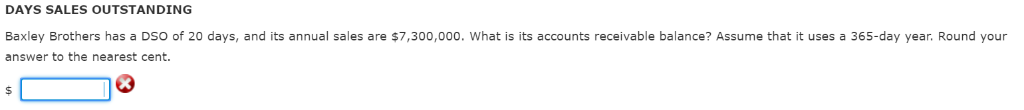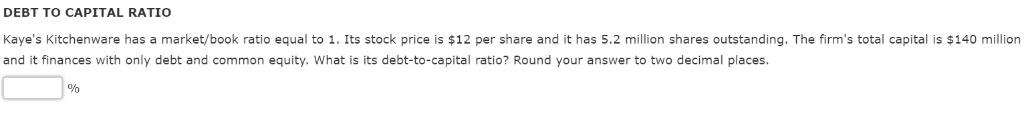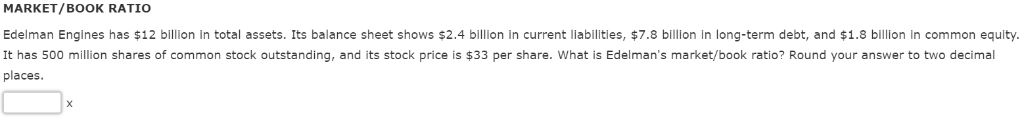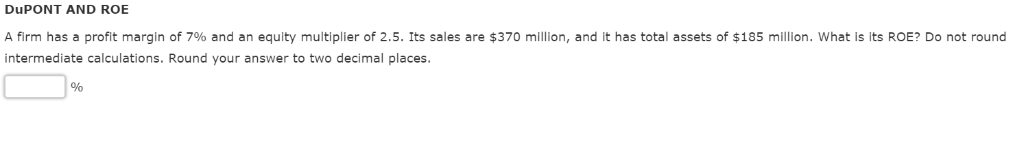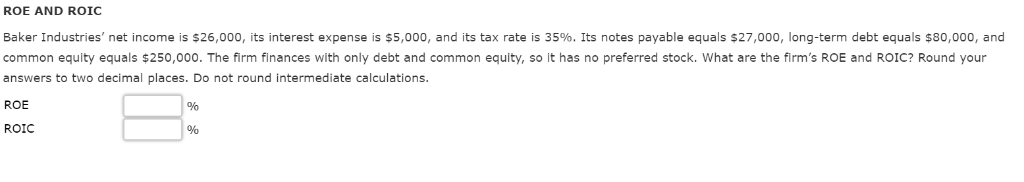DAYS SALES OUTSTANDING Baxley Brothers has a DSO of 20 days, and its annual sales are \$7,300,000. What is its accounts receivable balance? Assume that it uses a 365-day year. Round your answer to the nearest cent. DEBT TO CAPITAL RATIO Kaye&#39;s Kitchenware has a market/book ratio equal to 1. Its stock price is \$12 per share and it has 5.2 million shares outstanding. The firm&#39;s total capital is \$140 million and it finances with only debt and common equity. What is its debt-to-capital ratio? Round your answer to two decimal places MARKET/BOOK RATIO Edelman Engines has \$12 billion in total assets. Its balance sheet shows \$2.4 billion in current liabilities, \$7.8 billion in long-term debt, and \$1.8 billion in common equity. It has 500 million shares of common stock outstanding, and its stock price is \$33 per share. What is Edelman&#39;s market/book ratio? Round your answer to two decimal places. DuPONT AND ROE A firm has a profit margin of 7% and an equity multiplier of 2.5. Its sales are \$370 million, and it has total assets of \$185 million, what is its ROE? Do not round intermediate calculations. Round your answer to two decimal places. ROE AND ROIC Baker Industries, net income is \$26,000, its interest expense is \$5,000, and its tax rate is 35%. Its notes payable equals \$27,000, long-term debt equals \$80,000, and common equity equals \$250,000. The firm finances with only debt and common equity, so it has no preferred stock. What are the firm&#39;s ROE and ROIC? Round your answers to two decimal places. Do not round intermediate calculations ROE ROIC

manish jayant 22-Apr-2020Get solution